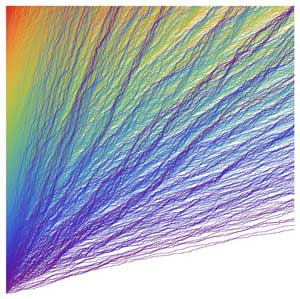# Stochastic Processes

Listed on this page are current research projects being offered for the Vacation Scholarship Program.

## Performance of a sensor network

(Also listed in Operations Research, posted for 2018-2019)

Wireless networks known as (ad hoc) sensor networks consist of sensing devices known as sensor nodes or sensors. Each sensor node collects information, which is then relayed back to a main station known as a base station or sink. Due to a limited transmission range, each node may need to relay the information through other nodes, resulting in multi-hop relaying. In other words, the collected information is relayed via a pathway of sensors rather than from each sensor directly to the sink. Furthermore, sensors may only operate for a limited time before becoming inoperative, thus needing to be repaired.

In this project we consider a mathematical model for a sensor network in which sensors communicate with the sink via a pathway or route, which is constructed with some simple routing rule, such as choosing the nearest neighbour. We will assume that sensors are operational for an exponentially distributed length of time with parameter λ, before being repaired in an exponentially distributed length of time with parameter μ.

We are interested in calculating performance measures such as the expected length of time until the network becomes disconnected (that is, at least one sensor cannot communicate with the base station), and the expected proportion of time the network is fully connected.

For this project, some familiarity with MATLAB or some other programming language (for example, R, Python, Julia etc) is desirable as initially the model will be simulated, before deriving some analytical results.

Contact: Mark Fackrell fackrell@unimelb.edu.au and Paul Keeler h.keeler@unimelb.edu.au

## Stochastic vertex models

(Also listed in Mathematical Physics & Statistical Mechanics, posted for 2018-2019)

Stochastic vertex models are a class of Markov processes in discrete two-dimensional space. In the simplest cases, they can be described in terms of paths which propagate randomly in a south-west to north-east direction, with an exclusion property that forbids two paths from overlapping. Stochastic vertex models have a rich scaling theory, under which certain observables can be shown to converge to solutions of stochastic partial differential equations, such as the Kardar--Parisi--Zhang (KPZ) equation. This project will serve as an introduction to these topics, with the aim of promoting further study in the area. Computer simulations (such as the one shown below) will be strongly encouraged.

Contact: Michael Wheeler, wheelerm@unimelb.edu.au